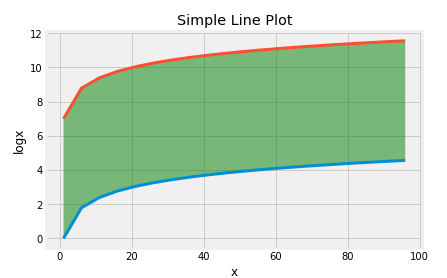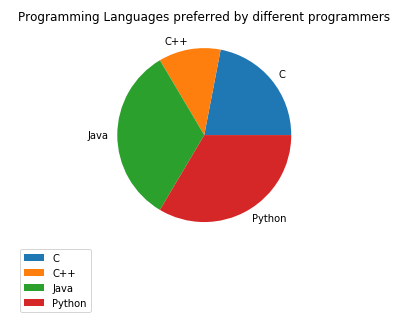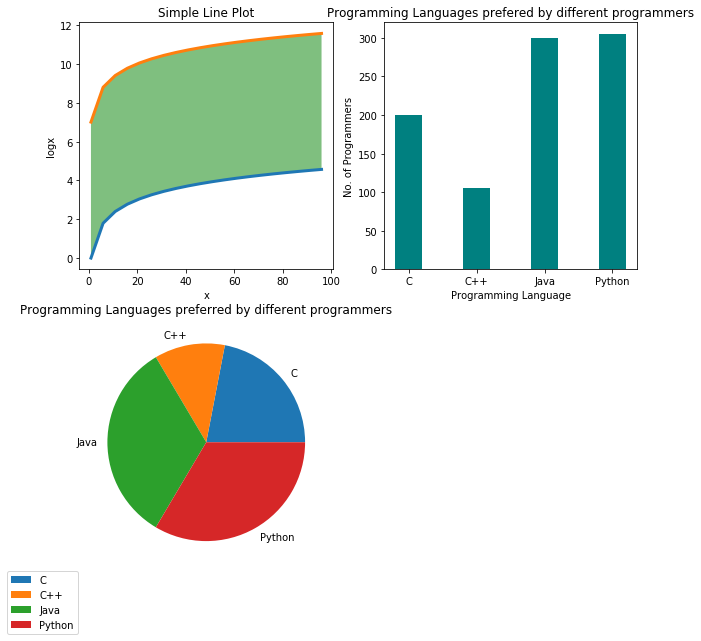# Easiest way to create Plots and Charts in Python | Matplotlib for Datascience and Machine Learning

Machine Learning and Data Science are two hot topic in the current tech world. People are easily attracted by these fancy topics. These are fascinating topics but are not easy to learn. Before you can excel in these stuffs you should master some prerequisites among which Mathematics and Data Visualization are the most important one. We will be talking about data visualization here.

Data Visualization have equal importance in Data Science and Machine learning. In data Science visual are use for analysis and presentation purpose. In Machine learning we use charts and plots to analyze relation between different parameters, see the training result and make learning algorithms. There are many data visualization library available. In this tutorial we are going with Matplotlib which is one of the most popular Python library for data visualization.REQUIREMENTS:

a. Python 3
b. Basic knowledge of graphs and plots
c. Jupyter Notebook (recommended)

In this tutorial we will be using Jupyter Notebookwith python which is highly recommended for any data science and Machine Learning works.

1. Simple Line Plots

Line plots are used to plot series of (x,y) cordinates in graph which may be connected by plain lines according to nature of plotted points. In matplotlib 'matplotlib.pyplot.plot' function is used for this.

```#library imports
import matplotlib.pyplot as plt
import numpy as np
import pandas as pd
%matplotlib inline```
```#simple plot
x=np.arange(1,100,5)
y=np.log(x)
print(y)
plt.plot(x,y)
plt.xlabel('x')
plt.ylabel('logx')
plt.title('Simple Line Plot')```
Here we plotted x vs log of x values. We can do multiple line plot in same graph with different colors. Let's add x vs y+7 plot too and fill the space between two ploot with color.So, our final code will look like this.
```x=np.arange(1,100,5)
y=np.log(x)
plt.plot(x,y,x,y+7,linewidth=3)
plt.xlabel('x')
plt.ylabel('logx')
plt.title('Simple Line Plot')
plt.fill_between(x,y,y+7,facecolor='g', alpha=0.5)```

plt.xlabel,plt.ylabel is used to set label on corresponding axes while plt.title set the title for entire plot.

And our final output will loke like this.#### 2. Bar Chart

Bar charts are used to represent categorical data with rectangular box. The width and height of bar is determined by corresponding data values.
Syntax in matplotlib
`` plt.bar(x, height, width=0.8, bottom=None, \*, align='center', data=None)``

Since Bar Chart is used to plot categorical data, let's create it using data stored in a python dictionary.

```data = {'C':200, 'C++':105, 'Java':300,'Python':305}
language = list(data.keys())
values = list(data.values())
plt.bar(language, values, color ='teal', width = 0.4)
plt.xlabel("Programming Language")
plt.ylabel("No. of Programmers")
plt.title("Programming Languages prefered by different programmers")
```

The output will look like below.

#### 3. Pie Chart

Pie chart is circular statistical graphic to represent proportional data with different slices. Pie chart generally shows the percentage ratio of categorical data. In matplotlib, pie graph is actually combination of different slices which proportion sums up to 360 degree. Here is the synta to create pie chart in matplotlib.

```plt.pie(data, explode=None, labels=None, colors=None,

Let us use the same programming language vs programmers data to plot pie chart.

```data = {'C':200, 'C++':105, 'Java':300,'Python':305}
language = list(data.keys())
values = list(data.values())
plt.pie(values,labels=language)
plt.title("Programming Languages preferred by different programmers")
plt.legend(labels=language,bbox_to_anchor=(0,0 ))
```

'plt.legend' is used to display Labels to know color representation and can be used with line plot and bar chart too.

The output of above code looks like below.#### Plot Styling and Decoration

There are various ways to make your plot look attractive. Here are few tips to make them look better.
The size of the figure can be changed as our need. The syntax to change figure size is:
`plt.figure(figsize=(x_size,y_size)) `

The sizes are in inches.

b. Use inbuilt theme and styles

There are many inbuilt styles and themes to decorate our plots. You can find the list of all the inbuilt style here. Style sheets reference

Here is an example on how styles can be applied in matplotlib figures.

`plt.style.use('seaborn-bright')#set the style of figure`
`theme = plt.get_cmap('hsv')# set color scheme `

c. Use subplot to display multiple charts in single figure

We can display multiple charts in a single figure in the form of grid. It is done in matplotlib using 'subplot' method. The syntax for subplot is
`subplot(nrows, ncols, index, **kwargs)`
All the above figure can be combined in a single figure using the code below.
```plt.figure(figsize=(10,10))
plt.subplot(2,2,1)
x=np.arange(1,100,5)
y=np.log(x)
z=np.exp(x)
plt.plot(x,y,x,y+7,linewidth=3)
plt.xlabel('x')
plt.ylabel('logx')
plt.title('Simple Line Plot')
plt.fill_between(x,y,y+7,facecolor='g', alpha=0.5)

plt.subplot(2,2,2)
data = {'C':200, 'C++':105, 'Java':300,'Python':305}
language = list(data.keys())
values = list(data.values())
plt.bar(language, values, color ='teal',
width = 0.4)
plt.xlabel("Programming Language")
plt.ylabel("No. of Programmers")
plt.title("Programming Languages prefered by different programmers")

plt.subplot(2,2,3)
data = {'C':200, 'C++':105, 'Java':300,'Python':305}
language = list(data.keys())
values = list(data.values())
plt.pie(values,labels=language)
plt.title("Programming Languages preferred by different programmers")
plt.legend(labels=language,bbox_to_anchor=(0,0 ))
```

The output looks like below.